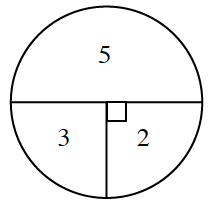### Home > GC > Chapter 11 > Lesson 11.1.2 > Problem11-23

11-23.

Marina needs to win $10$ tickets to get a giant stuffed panda bear. To win tickets, she throws a dart at the dartboard at right and wins the number of tickets listed in the region where her dart lands. Unfortunately, she only has enough money to play the game three times. If she throws the dart randomly, do you expect that she will be able to win enough tickets? Assume that each dart will land on the dartboard.Find the probability of a dart landing in each region.

$P\left(5\right)=\frac{1}{2}$

$P\left(3\right)=\frac{1}{4}$

$P\left(2\right)=\frac{1}{4}$

Calculate the expected value for one throw. Multiply the probability of each outcome times the amount that is earned. Total these possibilities.

$5\cdot\frac{1}{2}+3\cdot\frac{1}{4}+2\cdot\frac{1}{4}=3.75$

Calculate the expected value for $3$ throws.

$3·3.75=11.25$

It is expected that she will win $11.25$ tickets, so she should be able to get the panda bear.